# Power of 168 vs 618 (lexer)

Warning

This page is referring to child section which source is inherited by the prime spin with partitions listed below. Please return always to the Home Page to track the hierarchy.

You may learn that sets of algebraic objects has a multilinear relationship related to a vector space called tensor. Tensors may map between different objects such as vectors, scalars, even other tensors contained in a group of partitions.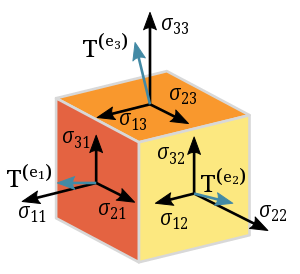In linear algebra, this vector is known as eigenvector, a nonzero vector that changes at most by a scalar factor when that linear transformation is applied to it. The corresponding eigenvalues is the factor by which the eigenvector is scaled.

Note

A 2×2 real and symmetric matrix representing a stretching and shearing of the plane. The eigenvectors of the matrix (red lines) are the two special directions such that every point on them will just slide on them (Wikipedia).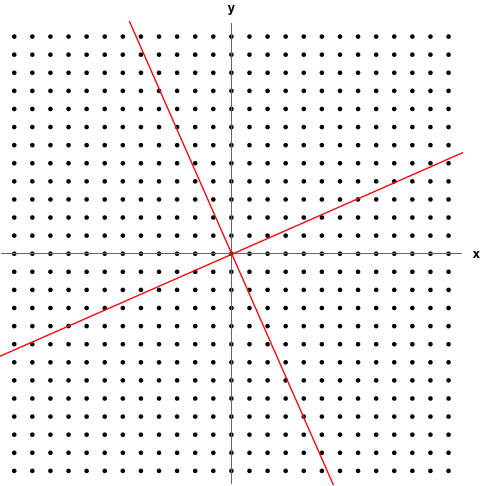It will be forced back to Δ19 making a cycle that bring back the 12 to → 13 of 9 collumns and replicate The Scheme 13:9 through (i=9,k=13)=9x3=27 with entry form of (100/50=2,60,40) as below: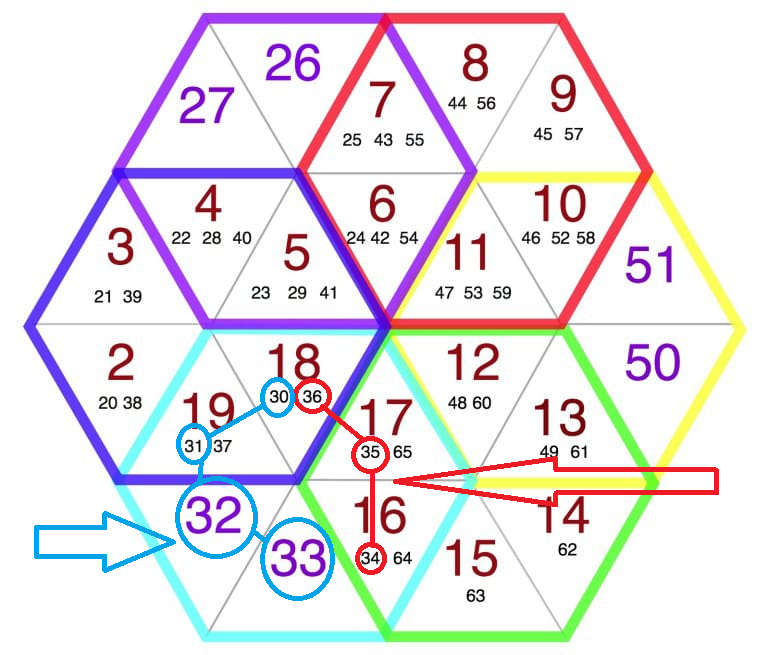You may see this scheme is build by 3 (three) layers where the next layer will continue the primes object by carrying the tensor of prime 31 and 71 of previous layer. So it will return to the beginning position within 60+40=100 nodes per layer.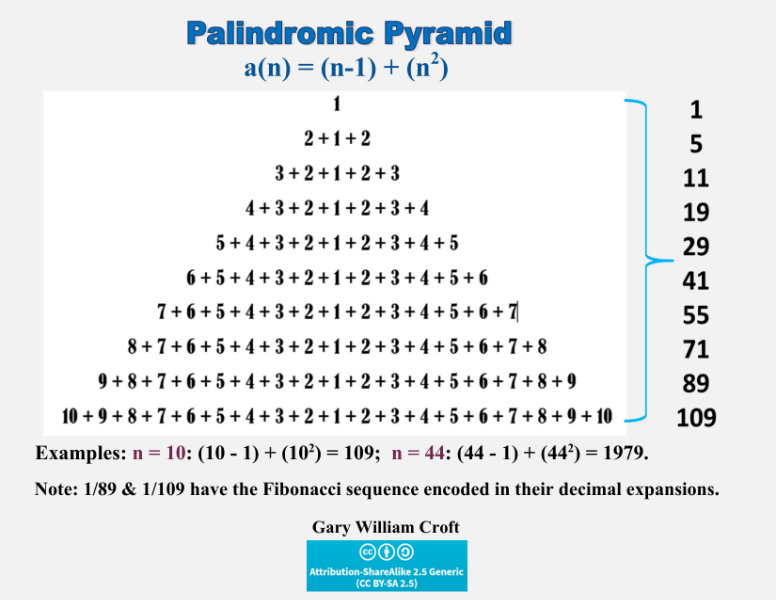By this project we are going to use a library called Chevrotain. It can be used to build Lexers, Parsers and Interpreters for various use cases ranging from simple config files to full fledged programming languages.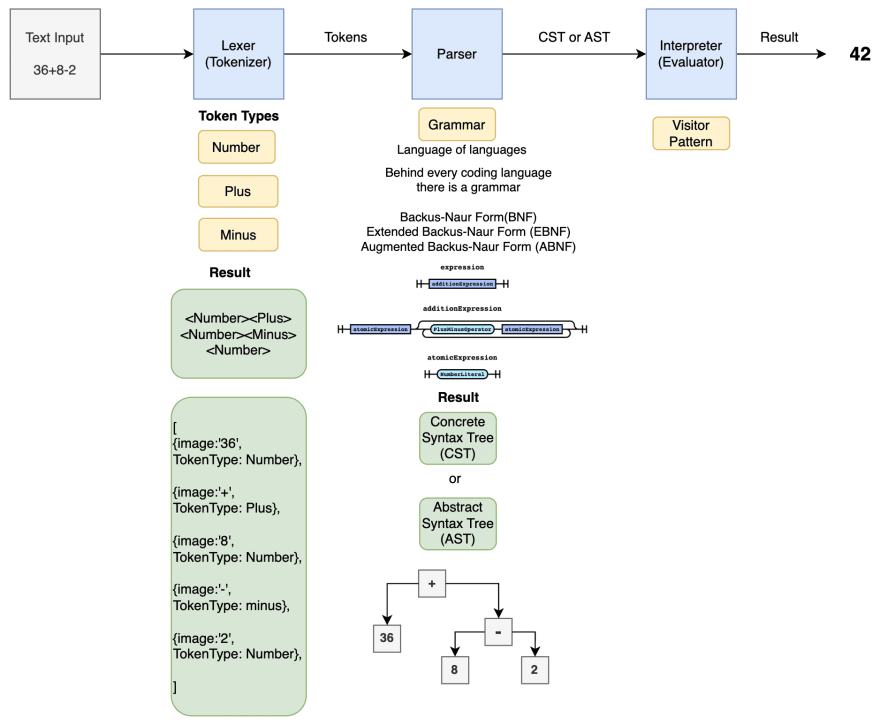The nodes are taken their place by a consideration that the 1st layer will have the same configuration as when the 3 (three) layers are combined. By this 1000 primes then 168-150=Δ18 is raised on the next turn.

Note

String theories require extra dimensions of spacetime for their mathematical consistency. In bosonic string theory, spacetime is 26-dimensional, while in superstring theory it is 10-dimensional, and in M-theory it is 11-dimensional (Wikipedia).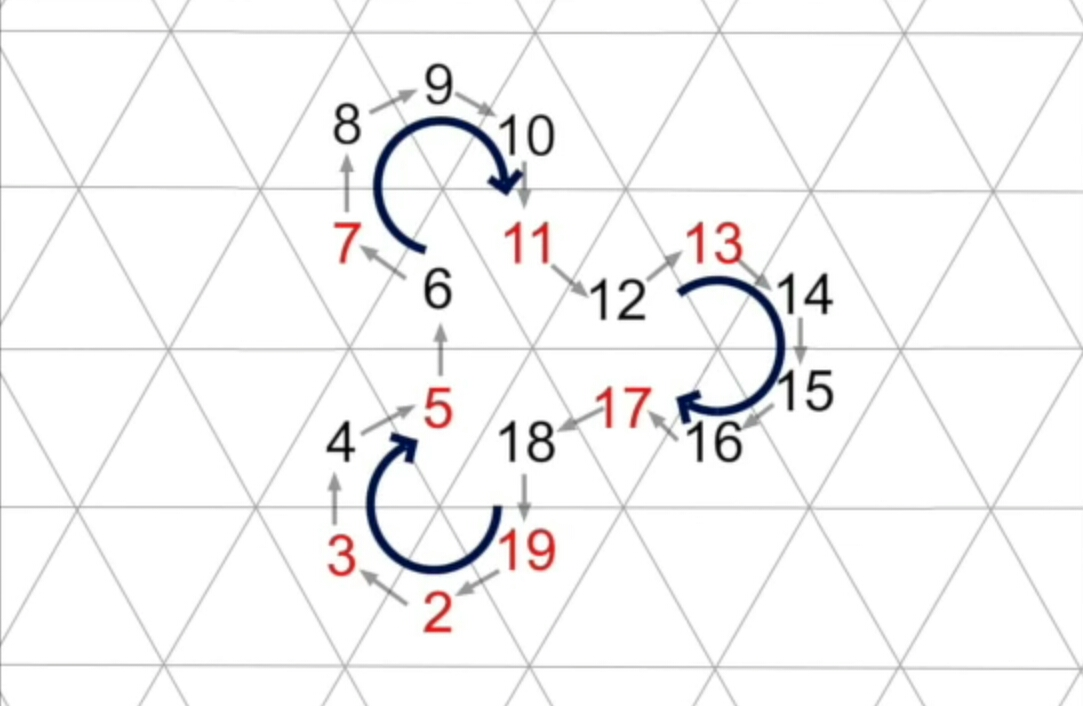Each result goes to the 9th object of prime 67 which is 19th prime. So when the cycle has passed the 10th object then the 43 objects will be laid by 9 collumns and slightly forming a recombination which facilitate them to finaly generate 1000 primes